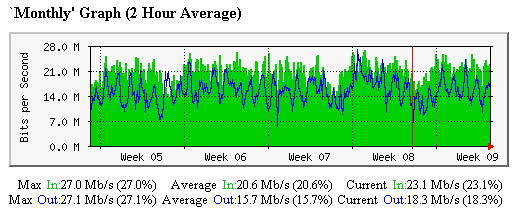# 95th Percentile Monitoring Explained

As if learning about Megabits-per-second wasn’t enough, yet another technical term pertaining to Colocation bandwidth that you’ve probably heard is 95th Percentile Monitoring – also called the 95% Method. So what does that mean? To illustrate, we’ll revisit the data that was graphed in the previous section, but this time we’ll look at the monthly graph, as opposed to the daily graph:As you can see, this graph, which is for the same Colocation network interface as the one shown previously, has the data rate on the vertical access, and the passage of time on the horizontal access. About five weeks work of data is graphed here. You’ll notice that the sample rate has been consolidated to two hour intervals for this graph – using smaller intervals does not make a statistically significant difference when graphing data over a time span this large.

So how would a Colocation service provider compute bandwidth usage for the month, using this data? You might assume that it would be fair to add the Average In and Average Out values (remember, Internet traffic travels in both directions) – a total of 36.3 Mbps in this case – and declare that to be the official usage for the month shown. In fact, many data centers will do just that. However, there is another way: the 95th Percentile Method. Here’s how it works:

• A log of 30 days worth of traffic samples at the default sample frequency (every 5 minutes is typical) is accumulated;
• The log is sorted in descending order, which places the biggest traffic peaks at the top;
• The top 5% of the log data is discarded;
• The value of the largest remaining peak becomes the effective bandwidth usage value for the month.

The advantage of the 95th Percentile method is that, over the course of a 30 day ‘month’ you can have up to 36 hours of peak traffic that will be completely ignored for billing purposes, no matter how high the peaks are. A secondary advantage is that the 95th percentile number is calculated only for the direction of greater flow, making the other direction free (as opposed to the average in + average out method). In the example of the monthly graph given above, the actual 95th Percentile traffic number for that data is 25.7 Mbps – 10.6 Mbps lower than using the ‘sum of the averages’ method.

As you can see, 95th Percentile Monitoring can be quite advantageous when getting Colocation bandwidth. There is one exception to this – in situations where there is normally very little bandwidth activity, but periodically large bursts of high traffic activity occur, 95th percentile could potentially work against you in the event that the cumulative bursts added up to more than 36 hours in the billing period. In practice this situation is uncommon, as nearly all carriers utilize the percentile method for billing of bandwidth, the best way to address this is to try to know (or predict) your usage profile and order service accordingly. One tactic: ordering a flat-rate bandwidth plan, as discussed in the next topic.